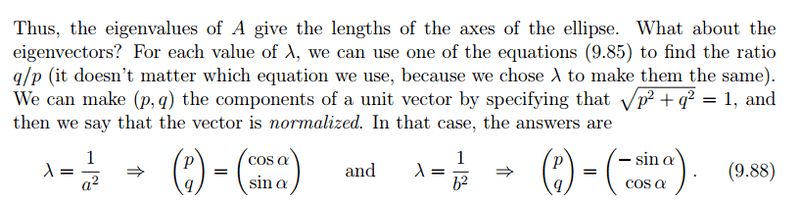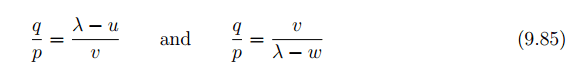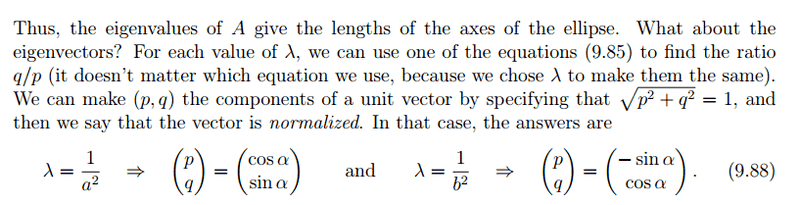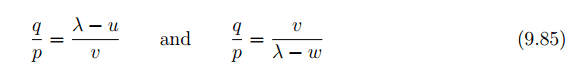# Finding the unit vector for an ellipse

• InclusiveMonk

## Homework Statement

Given the ellipse
##0.084x^2 − 0.079xy + 0.107y^2 = 1 ##
Find the semi-major and semi-minor axes of this ellipse, and a unit vector in the
direction of each axis.

I have calculated the semi-major and minor axes, I am just stuck on the final part.

## Homework Equationsthis is where ##ux^2+2vxy+wy^2=1##

## The Attempt at a Solution

I have calculated the two eigenvalues, 0.13664... and 0.05436... and therefore found the semi-major and semi-minor axes. I'm just not sure where to go next. I have worked out the ratios for q/p but I'm not sure how to use them.

## Homework Statement

Given the ellipse
##0.084x^2 − 0.079xy + 0.107y^2 = 1 ##
Find the semi-major and semi-minor axes of this ellipse, and a unit vector in the
direction of each axis.

I have calculated the semi-major and minor axes, I am just stuck on the final part.

## Homework Equationsthis is where ##ux^2+2vxy+wy^2=1##

## The Attempt at a Solution

I have calculated the two eigenvalues, 0.13664... and 0.05436... and therefore found the semi-major and semi-minor axes. I'm just not sure where to go next. I have worked out the ratios for q/p but I'm not sure how to use them.

What did you get for the semi-major and semi-minor axes? If you found the angle ##\alpha## that one of them makes with the x-axis, the vector ##<cos(\alpha), sin(\alpha)>## is a unit vector in the direction of one of these axes. ##<-sin(\alpha), cos(\alpha)>## is a unit vector in the other one's direction.

What did you get for the semi-major and semi-minor axes? If you found the angle ##\alpha## that one of them makes with the x-axis, the vector ##<cos(\alpha), sin(\alpha)>## is a unit vector in the direction of one of these axes. ##<-sin(\alpha), cos(\alpha)>## is a unit vector in the other one's direction.

I got 2.705 for the semi-minor and 4.2890 for the semi-major. As far as I was aware these were just lengths, I solved them by equating those two q/p equations and rearranging to give ##λ^2-(u+w)λ+uw-v^2=0## and solving.
I basically followed the steps on these notes http://quince.leeds.ac.uk/~phyjkp/Files/Teach/phys2370notes4.pdf [Broken] (It's page 2 on there) but I'm really confused about that last part (9.88)

Last edited by a moderator:
If you have u and v, you can use either eigenvalue and equation 9.85 to find the ratio q/p. Then the coordinates of a direction vector are (p, q).